# NCERT solutions for Class 7 Maths chapter 1 - Integers [Latest edition]

#### Chapters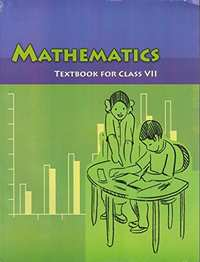## Chapter 1: Integers

Exercise 1.1Exercise 1.2Exercise 1.3Exercise 1.4
Exercise 1.1 [Pages 4 - 5]

### NCERT solutions for Class 7 Maths Chapter 1 IntegersExercise 1.1 [Pages 4 - 5]

Exercise 1.1 | Q 1.1 | Page 4

Following number line shows the temperature in degree celsius (°C) at different places on a particular day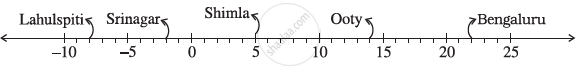Observe this number line and write the temperature of the places marked on it.

Exercise 1.1 | Q 1.2 | Page 4

Following number line shows the temperature in degree celsius (°C) at different places on a particular day.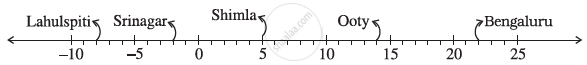What is the temperature difference between the hottest and the coldest places among the above?

Exercise 1.1 | Q 1.3 | Page 4

Following number line shows the temperature in degree celsius (°C) at different places on a particular day.What is the temperature difference between Lahulspiti and Srinagar?

Exercise 1.1 | Q 1.4 | Page 4

Following number line shows the temperature in degree celsius (°C) at different places on a particular day.Can we say temperature of Srinagar and Shimla taken together is less than the temperature at Shimla? Is it also less than the temperature at Srinagar?

Exercise 1.1 | Q 2 | Page 4

In a quiz, positive marks are given for correct answers and negative marks are given for incorrect answers. If jack’s scores in five successive rounds were 25, − 5, − 10, 15 and 10, what was his total at the end?

Exercise 1.1 | Q 3 | Page 4

At Srinagar temperature was − 5°C on Monday and then it dropped by 2°C on Tuesday. What was the temperature of Srinagar on Tuesday? On Wednesday, it rose by 4°C. What was the temperature on this day?

Exercise 1.1 | Q 4 | Page 4

A plane is flying at the height of 5000 m above the sea level. At a particular point, it is exactly above a submarine floating 1200 m below the sea level. What is the vertical distance between them?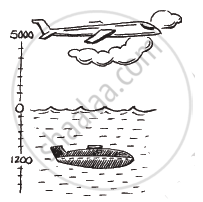Exercise 1.1 | Q 5 | Page 4

Mohan deposits Rs 2,000 in his bank account and withdraws Rs 1,642 from it, the next day. If withdrawal of amount from the account is represented by a negative integer, then how will you represent the amount deposited? Find the balance in Mohan’s account after the withdrawal.

Exercise 1.1 | Q 6 | Page 4

Rita goes 20 km towards east from a point A to the point B. From B, she moves 30 km towards west along the same road. If the distance towards east is represented by a positive integer then, how will you represent the distance travelled towards west? By which integer will you represent her final position from A?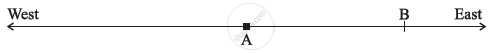Exercise 1.1 | Q 7 | Page 5

In a magic square each row, column and diagonal have the same sum. Check which of the following is a magic square.

1)

 5 -1 -4 -5 -2 7 0 3 -3

2)

 1 -10 0 -4 -3 -2 -6 4 -7

Exercise 1.1 | Q 8.1 | Page 5

Verify a − (−b) = a + b for the following values of a and ba = 21, b = 18

Exercise 1.1 | Q 8.2 | Page 5

Verify a − (−b) = a + b for the given values of a and b.

a = 118, b = 125

Exercise 1.1 | Q 8.3 | Page 5

Verify a − (−b) = a + b for the following values of a and b.

a = 75, b = 84

Exercise 1.1 | Q 8.4 | Page 5

Verify a − (−b) = a + b for the following values of a and b.

a = 28, b = 11

Exercise 1.1 | Q 9.1 | Page 5

Use the sign of >, < or = in the box to make the statements true.

(– 8) + (– 4)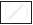(–8) – (– 4)

Exercise 1.1 | Q 9.2 | Page 5

Use the sign of >, < or = in the box to make the statements true.

(– 3) + 7 – (19)15 – 8 + (– 9)

Exercise 1.1 | Q 9.3 | Page 5

Use the sign of >, < or = in the box to make the statements true.

23 – 41 + 1123 – 41 – 11

Exercise 1.1 | Q 9.4 | Page 5

Use the sign of >, < or = in the box to make the statements true.

39 + (– 24) – (15)36 + (– 52) – (– 36)

Exercise 1.1 | Q 9.5 | Page 5

Use the sign of >, < or = in the box to make the statements true.

– 231 + 79 + 51–399 + 159 + 81

Exercise 1.1 | Q 10 | Page 5

A water tank has steps inside it. A monkey is sitting on the topmost step (i.e., the first step). The water level is at the ninth step.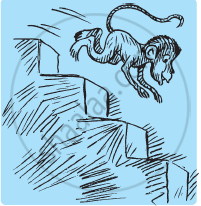1) He jumps 3 steps down and then jumps back 2 steps up. In how many jumps will he reach the water level?

2) After drinking water, he wants to go back. For this, he jumps 4 steps up and then jumps back 2 steps down in every move. In how many jumps will he reach back the top step?

3) If the number of steps moved down is represented by negative integers and the number of steps moved up by positive integers, represent his moves in part (i) and (ii) by completing the following; (a) − 3 + 2 − … = − 8 (b) 4 − 2 + … = 8. In (a) the sum (− 8) represents going down by eight steps. So, what will the sum 8 in (b) represent?

Exercise 1.2 [Page 9]

### NCERT solutions for Class 7 Maths Chapter 1 IntegersExercise 1.2 [Page 9]

Exercise 1.2 | Q 1.1 | Page 9

Write down the pair of integers whose sum is − 7

Exercise 1.2 | Q 1.2 | Page 9

Write down the pair of integers whose difference is − 10

Exercise 1.2 | Q 1.3 | Page 9

Write down the pair of integers whose: sum is 0

Exercise 1.2 | Q 2.1 | Page 9

Write a pair of negative integers whose difference gives 8.

Exercise 1.2 | Q 2.2 | Page 9

Write a negative integer and a positive integer whose sum is − 5.

Exercise 1.2 | Q 2.3 | Page 9

Write a negative integer and a positive integer whose difference is − 3.

Exercise 1.2 | Q 3 | Page 9

In a quiz, team A scored − 40, 10, 0 and team B scored 10, 0 − 40 in three successive rounds. Which team scored more? Can we say that we can add integers in any order?

Exercise 1.2 | Q 4.1 | Page 9

Fill in the blanks to make the following statements true:

(− 5) + (− 8) = (− 8) + (…)

Exercise 1.2 | Q 4.2 | Page 9

Fill in the blanks to make the following statements true:

− 53 + … = − 53

Exercise 1.2 | Q 4.3 | Page 9

Fill in the blanks to make the following statements true:

17 + … = 0

Exercise 1.2 | Q 4.4 | Page 9

Fill in the blanks to make the following statements true:

[13 + (− 12)] + (…) = 13 + [(− 12) + (− 7)]

Exercise 1.2 | Q 4.5 | Page 9

Fill in the blanks to make the following statements true:

(− 4) + [15 + (− 3)] = [(− 4) + 15] + .........

Exercise 1.3 [Pages 21 - 22]

### NCERT solutions for Class 7 Maths Chapter 1 IntegersExercise 1.3 [Pages 21 - 22]

Exercise 1.3 | Q 1.01 | Page 21

Find the following products: 3 × (− 1)

Exercise 1.3 | Q 1.02 | Page 21

Find the following products : (− 1) × 225

Exercise 1.3 | Q 1.03 | Page 21

Find the following products: (− 21) × (− 30)

Exercise 1.3 | Q 1.04 | Page 21

Find the following products:  (− 316) × (− 1)

Exercise 1.3 | Q 1.05 | Page 21

Find the following products: (− 15) × 0 × (−18)

Exercise 1.3 | Q 1.06 | Page 21

Find the following products: (− 12) × (− 11) × (10)

Exercise 1.3 | Q 1.07 | Page 21

Find the following products: 9 × (− 3) × (− 6)

Exercise 1.3 | Q 1.08 | Page 21

Find the following products: (− 18) × (− 5) × (− 4)

Exercise 1.3 | Q 1.09 | Page 21

Find the following products: (− 1) × (−2) × (− 3) × 4

Exercise 1.3 | Q 1.1 | Page 21

Find the following products: (− 3) × (− 6) × (− 2) × (− 1)

Exercise 1.3 | Q 2.1 | Page 21

Verify the following: 18 × [7 + (–3)] = [18 × 7] + [18 × (–3)]

Exercise 1.3 | Q 2.2 | Page 21

Verify the following: (–21) × [(– 4) + (– 6)] = [(–21) × (– 4)] + [(–21) × (– 6)]

Exercise 1.3 | Q 3.1 | Page 21

For any integer a, what is (− 1) × a equal to?

Exercise 1.3 | Q 3.2 | Page 21

Determine the integer whose product with (− 1) is (a) − 22 (b) 37 (c) 0

Exercise 1.3 | Q 4 | Page 21

Starting from (− 1) × 5, write various products showing some pattern to show (− 1) × (− 1) =1.

Exercise 1.3 | Q 5.1 | Page 21

Find the product, using suitable properties:

26 × (− 48) + (− 48) × (− 36)

Exercise 1.3 | Q 5.2 | Page 21

Find the product, using suitable properties:

8 × 53 × (−125)

Exercise 1.3 | Q 5.3 | Page 21

Find the product, using suitable properties:

15 × (− 25) × (− 4) × (− 10)

Exercise 1.3 | Q 5.4 | Page 21

Find the product, using suitable properties:

(− 41) × 102

Exercise 1.3 | Q 5.5 | Page 21

Find the product, using suitable properties:

625 × (− 35) + (− 625) × 65

Exercise 1.3 | Q 5.5 | Page 21

Find the product, using suitable properties:

625 × (− 35) + (− 625) × 65

Exercise 1.3 | Q 5.6 | Page 21

Find the product, using suitable properties:

7 × (50 − 2)

Exercise 1.3 | Q 5.7 | Page 21

Find the product, using suitable properties:

(− 17) × (− 29)

Exercise 1.3 | Q 5.8 | Page 21

Find the product, using suitable properties:

(− 57) × (− 19) + 57

Exercise 1.3 | Q 6 | Page 21

A certain freezing process requires that room temperature be lowered from 40°C at the rate of 5°C every hour. What will be the room temperature 10 hours after the process begins?

Exercise 1.3 | Q 7 | Page 21

In a class test containing 10 questions, 5 marks are awarded for every correct answer and (− 2) marks are awarded for every incorrect answer and 0 for questions not attempted.

1) Mohan gets four correct and six incorrect answers. What is his score?

2) Reshma gets five correct answers and five incorrect answers, what is her score?

3) Heena gets two correct and five incorrect answers out of seven questions she attempts. What is her score?

Exercise 1.3 | Q 8 | Page 21

A cement company earns a profit of Rs 8 per bag of white cement sold and a loss of Rs 5 per bag of grey cement sold

1) The company sells 3, 000 bags of white cement and 5,000 bags of grey cement in a month. What is its profit or loss?

2) What is the number of white cement bags it must sell to have neither profit nor loss, if the number of grey bags sold is 6,400 bags.

Exercise 1.3 | Q 9.1 | Page 22

Replace the blank with an integer to make it a true statement

(–3) × _____ = 27

Exercise 1.3 | Q 9.2 | Page 22

Replace the blank with an integer to make it a true statement.

5 × _____ = –35

Exercise 1.3 | Q 9.3 | Page 22

Replace the blank with an integer to make it a true statement.

_____ × (– 8) = –56

Exercise 1.3 | Q 9.4 | Page 22

Replace the blank with an integer to make it a true statement.

_____ × (–12) = 132

Exercise 1.4 [Page 26]

### NCERT solutions for Class 7 Maths Chapter 1 IntegersExercise 1.4 [Page 26]

Exercise 1.4 | Q 1.1 | Page 26

Evaluate the following: (–30) ÷ 10

Exercise 1.4 | Q 1.2 | Page 26

Evaluate the following: 50 ÷ (–5)

Exercise 1.4 | Q 1.3 | Page 26

Evaluate the following : (–36) ÷ (–9)

Exercise 1.4 | Q 1.4 | Page 26

Evaluate the following: (– 49) ÷ (49)

Exercise 1.4 | Q 1.5 | Page 26

Evaluate the following: 13 ÷ [(–2) + 1]

Exercise 1.4 | Q 1.6 | Page 26

Evaluate the following:0 ÷ (–12)

Exercise 1.4 | Q 1.7 | Page 26

Evaluate the following: (–31) ÷ [(–30) + (–1)]

Exercise 1.4 | Q 1.8 | Page 26

Evaluate the following: [(–36) ÷ 12] ÷ 3

Exercise 1.4 | Q 1.9 | Page 26

Evaluate the following: [(– 6) + 5)] ÷ [(–2) + 1]

Exercise 1.4 | Q 2.1 | Page 26

Verify that a ÷ (b + c) ≠ (a ÷ b) + (a ÷ c) for each of the following values of a, b and c.

a = 12, b = −4, c = 2

Exercise 1.4 | Q 2.2 | Page 26

Verify that a ÷ (b + c) ≠ (a ÷ b) + (a ÷ c) for each of the following values of a, b and c.

a = (− 10), b = 1, c = 1

Exercise 1.4 | Q 3.1 | Page 26

Fill in the blanks:

369 ÷ _____ = 369

Exercise 1.4 | Q 3.2 | Page 26

Fill in the blanks:

(–75) ÷ _____ = –1

Exercise 1.4 | Q 3.3 | Page 26

Fill in the blanks:

(–206) ÷ _____ = 1

Exercise 1.4 | Q 3.4 | Page 26

Fill in the blanks:

– 87 ÷ _____ = 87

Exercise 1.4 | Q 3.5 | Page 26

Fill in the blanks

_____ ÷ 1 = – 87

Exercise 1.4 | Q 3.6 | Page 26

Fill in the blanks:

_____ ÷ 48 = –1

Exercise 1.4 | Q 3.7 | Page 26

Fill in the blanks:

20 ÷ _____ = –2

Exercise 1.4 | Q 3.8 | Page 26

Fill in the blanks:

_____ ÷ (4) = –3

Exercise 1.4 | Q 4 | Page 26

Write five pairs of integers (ab) such a ÷ b = − 3. One such pair is (6, − 2) because 6 ÷ (− 2) = (− 3).

Exercise 1.4 | Q 5 | Page 26

The temperature at 12 noon was 10°C above zero. If it decreases at the rate of 2°C per hour until midnight, at what time would the temperature be 8°C below zero? What would be the temperature at mid-night?

Exercise 1.4 | Q 6 | Page 26

In a class test (+ 3) marks are given for every correct answer and (−2) marks are given for every incorrect answer and no marks for not attempting any question. (i) Radhika scored 20 marks. If she has got 12 correct answers, how many questions has she attempted incorrectly? (ii) Mohini scores − 5 marks in this test, though she has got 7 correct answers. How many questions has she attempted incorrectly? (iii) Rakesh scores 18 marks by attempting 16 questions. How many questions has he attempted correctly and how many has he attempted incorrectly?

Exercise 1.4 | Q 7 | Page 26

An elevator descends into a mine shaft at the rate of 6 m/min. If the descent starts from 10 m above the ground level, how long will it take to reach − 350 m.

## Chapter 1: Integers

Exercise 1.1Exercise 1.2Exercise 1.3Exercise 1.4## NCERT solutions for Class 7 Maths chapter 1 - Integers

NCERT solutions for Class 7 Maths chapter 1 (Integers) include all questions with solution and detail explanation. This will clear students doubts about any question and improve application skills while preparing for board exams. The detailed, step-by-step solutions will help you understand the concepts better and clear your confusions, if any. Shaalaa.com has the CBSE Class 7 Maths solutions in a manner that help students grasp basic concepts better and faster.

Further, we at Shaalaa.com provide such solutions so that students can prepare for written exams. NCERT textbook solutions can be a core help for self-study and acts as a perfect self-help guidance for students.

Concepts covered in Class 7 Maths chapter 1 Integers are Associative Property of Multiplication of Integers, Concept for Natural Numbers, Concept for Whole Numbers, Concept of Negative Numbers, Concept of Integers, Representation of Integers on the Number Line, Concept for Ordering of Integers, Addition of Integers, Addition of Integers on Number line, Subtraction of Integers, Properties of Addition and Subtraction of Integers, Multiplication of a Positive and a Negative Integers, Multiplication of Two Negative Integers, Product of Three Or More Negative Integers, Closure Property of Multiplication of Integers, Commutative Property of Multiplication of Integers, Making Multiplication Easier, Division of Integers, Properties of Division of Integers, Distributive Property of Multiplication of Integers, Multiplication of Integers with Zero, Multiplicative Identity of Integers.

Using NCERT Class 7 solutions Integers exercise by students are an easy way to prepare for the exams, as they involve solutions arranged chapter-wise also page wise. The questions involved in NCERT Solutions are important questions that can be asked in the final exam. Maximum students of CBSE Class 7 prefer NCERT Textbook Solutions to score more in exam.

Get the free view of chapter 1 Integers Class 7 extra questions for Class 7 Maths and can use Shaalaa.com to keep it handy for your exam preparation